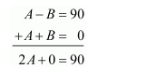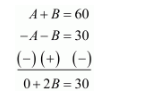# If tan (A−B)=13√ and tan (A+B)=3–√, 0° < A+B≤90°, A>B find A and B.

Question:

If $\tan (A-B)=\frac{1}{\sqrt{3}}$ and $\tan (A+B)=\sqrt{3}, 0^{\circ}B$ find $\mathrm{A}$ and $\mathrm{B}$.

Solution:

Given:

$\tan (A-B)=\frac{1}{\sqrt{3}}$......(1)

$\tan (A+B)=\sqrt{3}$......(2)

We know that,

$\tan 30^{\circ}=\frac{1}{\sqrt{3}}$.....(3)

$\tan 60^{\circ}=\sqrt{3}$......(4)

Now by comparing equation (1) and (3)

We get,

$A-B=30$....(5)

Now by comparing equation (2) and (4)

We get,

$A+B=60 \ldots \ldots(6)$

Now to get the values of A and B, let us solve equation (5) and (6) simultaneously

Therefore by adding equation (5) and (6)

We get,Therefore,

$2 A=90$

$\Rightarrow \quad A=\frac{90}{2}$

$\Rightarrow \quad A=45^{\circ}$

Hence $A=45^{\circ}$

Now by subtracting equation (5) from equation (6)

We get,Therefore,

$2 B=30$

$\Rightarrow B=\frac{30}{2}$

$\Rightarrow B=15^{\circ}$

Hence $B=15^{\circ}$

Therefore the values of A and B are as follows

$A=45^{\circ}$ and $B=15^{\circ}$